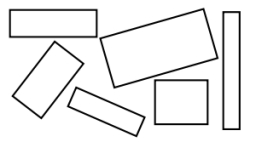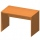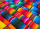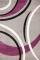# Mason 3

Mason has a rectangular garden that is 2/3 yard wide by 7/4 yards long. What is the area of Mason’s garden? Use a drawing to show your work.

A =  1.1667 yd2

### Step-by-step explanation:Did you find an error or inaccuracy? Feel free to write us. Thank you!Tips to related online calculators
Need help to calculate sum, simplify or multiply fractions? Try our fraction calculator.

#### You need to know the following knowledge to solve this word math problem:

We encourage you to watch this tutorial video on this math problem:

## Related math problems and questions:

• JuilaJuila has a rectangular table that is 3 1/2 feet long and 1 3/4 feet wide. What is the area of Juila's table?
• AlexandraAlexandra made a rectangular quilt the measured 3 1/4. 2 3/4 feet in width. What is the area of the quilt in square feet? Write an equation to solve.
• Father's gardenJohn's father has a garden in the back yard. The dimensions of the garden are shown here. A green rectangle is shown. The width is labeled as 2 and two-thirds feet. The length is labeled as 7 and three-fourths feet. Calculate the area of the garden. You f
• Long and wideWhat is the area of a rectangle that is 1 1/4 meters long and 3/4​ of a meter wide
• JoanneJoanne and Roger are planting a rectangular garden. The garden is 8 1/2 ft by 13 ft. They want to use half of the garden for cucumbers and half of the garden for tomatoes. They decide to separate the garden into two right triangles. What is the area of th
• A rectangle 6A rectangle measures 2 4/5 inches by 2 1/5 inches. What is its area?3 3/4 + 2 3/5 + 5 1/2 Show your solution.
• Little 2Little fields farm lies on a rectangular plot of land that measures 2 2/4 miles in length and 2 2/3 miles in width. What is it's area in square miles?
• Joy hasJoy has 3/4 yards of fabric. She cuts the fabric into pieces that are each 1/8 yard long. How many pieces of fabric does Joy have?
• Living room 3Pearl wants to buy carpet to cover her whole living room, except for the tiled floor. The tiled floor is 7 1/2 ft by 3 1/4 ft. Find the area the carpet needs to cover.
• A triangle 2A triangle has a base of 2 inches and a height of 2 3/4 inches. What is the area of the triangle?
• Area of a triangleWhat is the area of a triangle that has the base 4 1/4 and the height of 3 3/3?
• Swimming pool 5A rectangular inflatable swimming pool is 3 yards long, 2 5/8 yards wide, and  1 3/5 yard tall. What is the volume of the pool? Round to the nearest tenth.
• Numbers divisionWith what number should be divided mixed number 2 3/4 to get 11/12?
• Simplify 7Simplify. 7-1/3÷ 3-2/3 of 2+ 4-1/2÷ 2-1/4+ 1/2 solution and by step by step
• Rectangle areaWhat is the area of a rectangle that has a length of 4 feet and a width of 4/10 feet?
• Equivalent fractions 2Write the equivalent multiplication expression. 2 1/6÷3/4